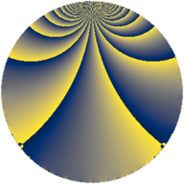# Properties

 Label 154.2.kLevel $154$ Weight $2$ Character orbit 154.k Rep. character $\chi_{154}(13,\cdot)$ Character field $\Q(\zeta_{10})$ Dimension $32$ Newform subspaces $1$ Sturm bound $48$ Trace bound $0$

# Related objects

## Defining parameters

 Level: $$N$$ $$=$$ $$154 = 2 \cdot 7 \cdot 11$$ Weight: $$k$$ $$=$$ $$2$$ Character orbit: $$[\chi]$$ $$=$$ 154.k (of order $$10$$ and degree $$4$$) Character conductor: $$\operatorname{cond}(\chi)$$ $$=$$ $$77$$ Character field: $$\Q(\zeta_{10})$$ Newform subspaces: $$1$$ Sturm bound: $$48$$ Trace bound: $$0$$

## Dimensions

The following table gives the dimensions of various subspaces of $$M_{2}(154, [\chi])$$.

Total New Old
Modular forms 112 32 80
Cusp forms 80 32 48
Eisenstein series 32 0 32

## Trace form

 $$32q + 8q^{4} + 10q^{7} - 4q^{9} + O(q^{10})$$ $$32q + 8q^{4} + 10q^{7} - 4q^{9} - 4q^{11} + 2q^{14} - 24q^{15} - 8q^{16} + 4q^{22} - 32q^{23} + 20q^{25} - 10q^{28} - 20q^{29} - 60q^{35} - 16q^{36} + 8q^{37} - 20q^{39} + 14q^{42} - 16q^{44} - 60q^{51} + 16q^{53} + 8q^{56} + 80q^{57} - 56q^{58} - 16q^{60} + 40q^{63} + 8q^{64} - 16q^{67} + 16q^{70} + 104q^{71} + 40q^{72} + 40q^{74} - 4q^{77} + 60q^{79} + 60q^{81} + 20q^{84} - 80q^{85} + 44q^{86} + 16q^{88} + 44q^{91} - 28q^{92} + 32q^{93} + 100q^{95} - 68q^{99} + O(q^{100})$$

## Decomposition of $$S_{2}^{\mathrm{new}}(154, [\chi])$$ into newform subspaces

Label Dim. $$A$$ Field CM Traces $q$-expansion
$$a_2$$ $$a_3$$ $$a_5$$ $$a_7$$
154.2.k.a $$32$$ $$1.230$$ None $$0$$ $$0$$ $$0$$ $$10$$

## Decomposition of $$S_{2}^{\mathrm{old}}(154, [\chi])$$ into lower level spaces

$$S_{2}^{\mathrm{old}}(154, [\chi]) \cong$$ $$S_{2}^{\mathrm{new}}(77, [\chi])$$$$^{\oplus 2}$$